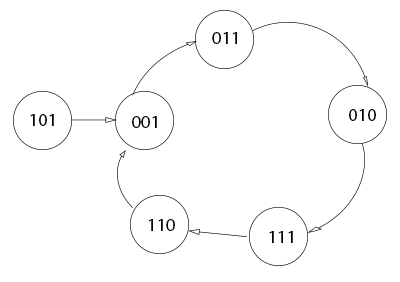### Exam Year

Tribhuvan University

Institute of Science and Technology

2074

Bachelor Level / first-semester / Science

Computer Science and Information Technology( CSC111 )

Digital Logic

Full Marks: 60 + 20 + 20

Pass Marks: 24 + 8 + 8

Time: 3 Hours

Candidates are required to give their answers in their own words as far as practicable.

The figures in the margin indicate full marks.

Group A

Attempts any two Questions?

1

Implement the following Functions F= Σ (0,3,5,6,7) using

1. Decoder
2. Multiplexer
3. PLA

2

Differentiate between PAL and PLA. Design a counter as shown in the state diagram below3

Draw a block diagram, truth table and logic circuit of 1*16 Demultiplexer and explain its working principle.

Group B

Attempts any eight questions

4

Perform the arithmetic operation (+42)+(-13) and (-42)-(-13) in binary using the signed -2’s-complement representation for negative numbers.

5

Express the complement of the following function in sum of min-terms.

F(A, B, C, D) = Σ(0, 2, 6, 11, 13, 14)

6

Reduce the following function using k-map F = wxy + yz + xy’z + x’y

7

Design a combinational circuit with three inputs and six outputs. The output binary number should be the square of the input binary number.

8

Design a 5 x 32 decoder with four 3 x 8 decoder with enable and one  2 x 4 decoder. Use block diagrams only.

9

Design and explain the Decimal adder with truth table and suitable diagram.

10

Explain shift register with parallel load. Highlight on its practical implications.

11

Explain master slave J-K flipflop.

12

Write short notes on (any two):

1. State diagram
2. De-Morgan’s theorem
3. TTL